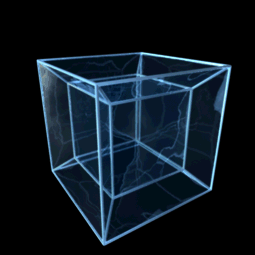# The Hyper Cube

“A point is a hypercube of dimension zero. If one moves this point one unit length, it will sweep out a line segment, which is a unit hypercube of dimension one. If one moves this line segment its length in a perpendicular direction from itself; it sweeps out a two-dimensional square. If one moves the square one unit length in the direction perpendicular to the plane it lies on, it will generate a three-dimensional cube. This can be generalized to any number of dimensions. For example, if one moves the cube one unit length into the fourth dimension, it generates a 4-dimensional unit hypercube.” – Wikipedia:hypercube

We can not literally represent the rotation of a hypercube in three dimensions. In this animation we see a two dimensional rendering of a three dimensional representation of fourth dimensional rotation.

As you watch the animation try to image that the hypercube is not changing shape at all, but that you are revolving around it through four dimensions.

Slightly distorted as due to my strict format.

Source